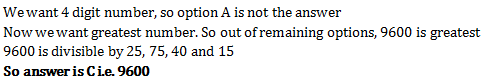# Aptitude Test for Freshers - Set 3

1)   If a:b = 5:7 and c:d = 2a:3b, then ac : bd is

a. 20:38
b. 50:147
c. 10:21
d. 50:151
 Answer  Explanation ANSWER: 50:147 Explanation: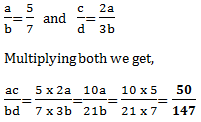2)   If A:B = 2:3, B:C = 4:5 and C:D = 6:7, then A:B:C:D is

a. 18:24:30:35
b. 16:24:30:35
c. 16:22:30:35
d. 16:24:15:35
 Answer  Explanation ANSWER: 16:24:30:35 Explanation: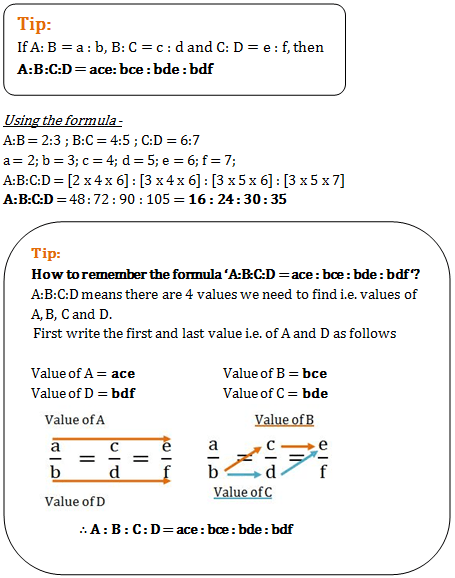3)   The three numbers are in the ratio 1/2 : 2/3 : 3/4. The difference between greatest and smallest numbers is 36. Find the numbers.

a. 72, 84, 108
b. 60, 72, 96
c. 72, 84, 96
d. 72, 96, 108
 Answer  Explanation ANSWER: 72, 84, 108 Explanation: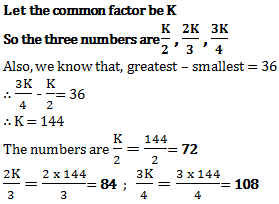4)   The ratio of numbers of girls and boys participating in sports of a school is 4:5. If the number of girls is 212, determine the number of boys participating in the sports.

a. 256
b. 265
c. 251
d. 263
 Answer  Explanation ANSWER: 265 Explanation: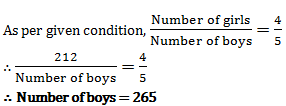5)   The ratio of market prices of wheat and paddy is 2:3 and the ratio of quantities consumed in a family is 5:4. Find the ratio of expenditure of wheat and paddy.

a. 6:5
b. 5:6
c. 1:1
d. 8:15
 Answer  Explanation ANSWER: 5:6 Explanation: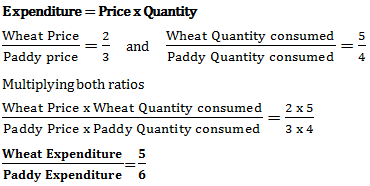6)   A cistern is normally filled with water in 10 hours but takes 5 hours longer to fill because of a leak in its bottom. If the cistern is full, the leak will empty the cistern in

a. 20 hours
b. 40 hours
c. 50 hours
d. 30 hours
 Answer  Explanation ANSWER: 30 hours Explanation: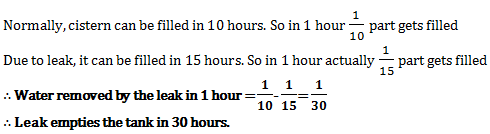7)   A and B can finish a piece of work in 30 days, B and C can finish it in 15 days, C and A in 10 days. Time taken by them together to do this work is?

a. 5 days
b. 2(1/2) days
c. 7(1/2) days
d. 10 days
 Answer  Explanation ANSWER: 10 days Explanation: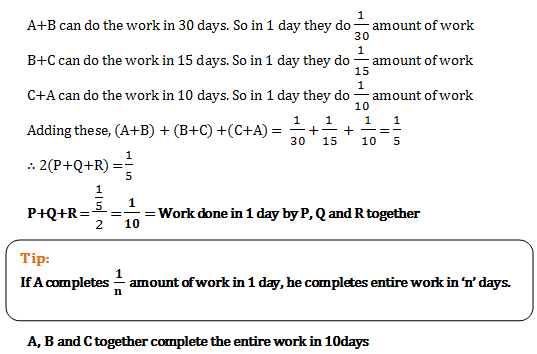8)   A can do 1/4th part of work in 10 days and B can do 40% work in 40 days  and C can do 1/3rd of the work in 13 days. Who will complete the work first?

a. A
b. B
c. C
d. A and C both
 Answer  Explanation ANSWER: C Explanation: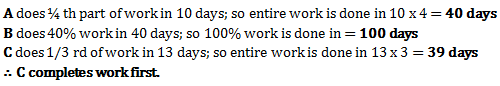9)   A takes twice as much time as B or thrice as much time as C to finish a piece of work; working together they can finish the work in 2 days. B can do the work alone in

a. 12 days
b. 4 days
c. 8 days
d. 6 days
 Answer  Explanation ANSWER: 6 days Explanation: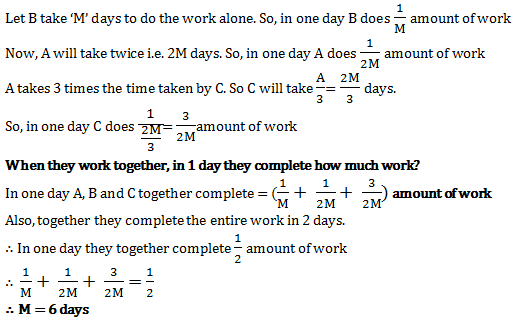10)   A circular wire of radius 21cm is cut and bent to form in the form of a rectangle whose sides are in the ratio of 6:5. Assuming, Π = 22/7, the area enclosed by the rectangle is

a. 540 cm2
b. 1080 cm2
c. 2160 cm2
d. 4320 cm2
 Answer  Explanation ANSWER: 1080 cm2 Explanation: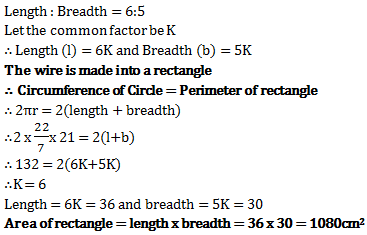11)   If the areas of the three adjacent faces of a cuboidal box are 120cm2, 72cm2 and 60cm2 respectively, find the volume of the box.

a. 7200 cm3
b. 720 cm3
c. 864 cm3
d. (72)2 cm3
 Answer  Explanation ANSWER: 720 cm3 Explanation: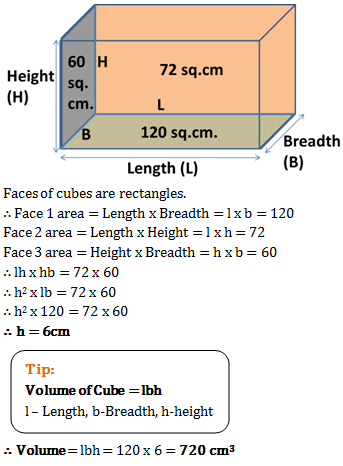12)   The number of coins of radius 0.75 cm and thickness 0.2 cm to be melted to make a right circular cylinder of height 8 cm and base radius 3 cm is

a. 640
b. 460
c. 500
d. 600
 Answer  Explanation ANSWER: 640 Explanation: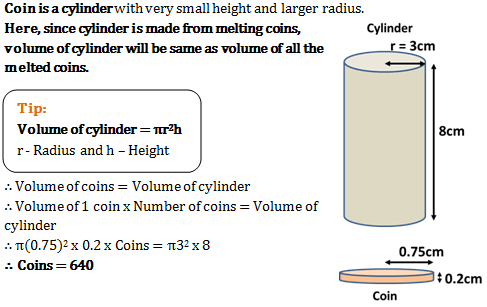13)   The area of the base of a right circular cone is 154cm2 and its height is 14cm. Taking π = 22/7, the curved surface of the cone is

a. 154 x 5 cm2
b. 154 x 7 cm2
c. 11 cm2
d. 5324 cm2
 Answer  Explanation ANSWER: 154 x 5 cm2 Explanation: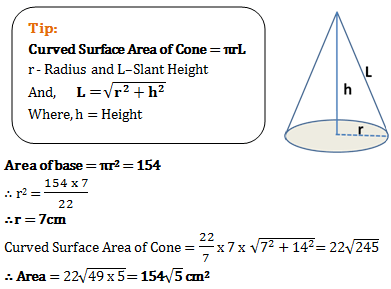14)   The L.C.M. of two numbers is 14560 and their H.C.F. is 13. If one of them is 416, the other is

a. 460
b. 455
c. 450
d. 445
 Answer  Explanation ANSWER: 455 Explanation: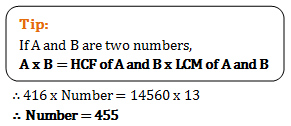15)   The sum of two numbers is 156 and their HCF is 13. The numbers of such number pairs is

a. 2
b. 5
c. 4
d. 3
 Answer  Explanation ANSWER: 2 Explanation: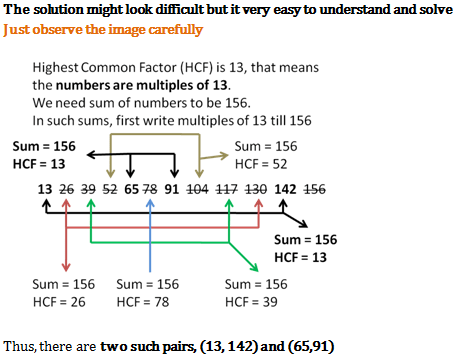16)   When a number is divided by 893 the remainder is 193. What will be the remainder when it is divided by 47?

a. 19
b. 5
c. 33
d. 23
 Answer  Explanation ANSWER: 5 Explanation: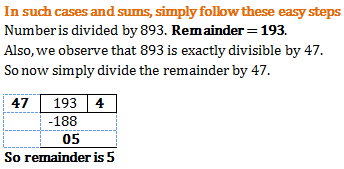17)   What is the least number which when divided by the numbers 3, 5, 6, 8, 10 and 12 leaves in each case a remainder 2 but which when divided by 13 leaves no remainder?

a. 312
b. 962
c. 1586
d. 1562
 Answer  Explanation ANSWER: 1586 Explanation: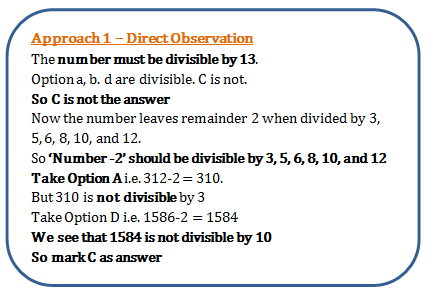18)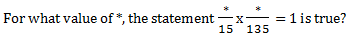a. 15
b. 25
c. 45
d. 35
 Answer  Explanation ANSWER: 45 Explanation: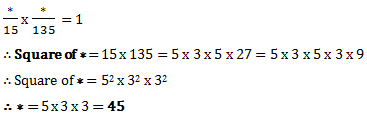19)   An integer is 10 more than its one-third part. The integer is

a. 15
b. 12
c. 18
d. 25
 Answer  Explanation ANSWER: 15 Explanation: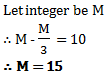20)   The greatest number of four digits which is divisible by 15, 25, 40, 75 is

a. 600
b. 9000
c. 9600
d. 9400
 Answer  Explanation ANSWER: 9600 Explanation: